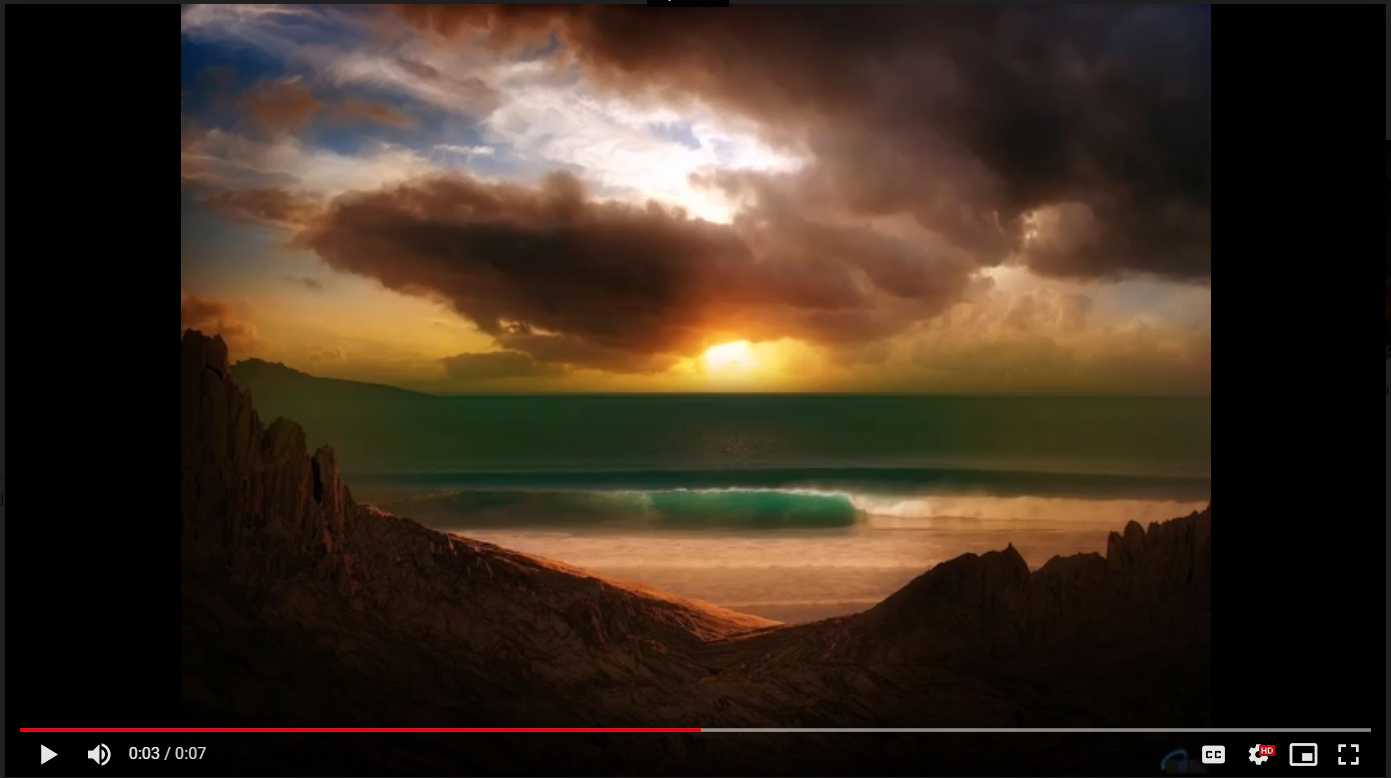# Python | Create video using multiple images using OpenCV

As we know OpenCV is a widely used library for image processing. It provides a wide sense of image processing. Let’s see how to create video using multiple images using OpenCV.

Install the following libraries:

PIL
cv2

Also, check the path before running the code otherwise you will be full with errors.

How it works ?
Using PIL library we are opening images and resizing them to their mean_height and mean_width because the video which will be created using cv2 library required the input images of same height and width.
Resized images are included in an array and frame of video is set with the mean_height and mean_width. Then by looping, we are appending each image to that frame.

Below is the implementation :

 `# importing libraries ` `import` `os ` `import` `cv2  ` `from` `PIL ``import` `Image  ` ` `  `# Checking the current directory path ` `print``(os.getcwd())  ` ` `  `# Folder which contains all the images ` `# from which video is to be generated ` `os.chdir(``"C:\\Python\\Geekfolder2"``)   ` `path ``=` `"C:\\Python\\Geekfolder2"` ` `  `mean_height ``=` `0` `mean_width ``=` `0` ` `  `num_of_images ``=` `len``(os.listdir(``'.'``)) ` `# print(num_of_images) ` ` `  `for` `file` `in` `os.listdir(``'.'``): ` `    ``im ``=` `Image.``open``(os.path.join(path, ``file``)) ` `    ``width, height ``=` `im.size ` `    ``mean_width ``+``=` `width ` `    ``mean_height ``+``=` `height ` `    ``# im.show()   # uncomment this for displaying the image ` ` `  `# Finding the mean height and width of all images. ` `# This is required because the video frame needs ` `# to be set with same width and height. Otherwise ` `# images not equal to that width height will not get  ` `# embedded into the video ` `mean_width ``=` `int``(mean_width ``/` `num_of_images) ` `mean_height ``=` `int``(mean_height ``/` `num_of_images) ` ` `  `# print(mean_height) ` `# print(mean_width) ` ` `  `# Resizing of the images to give ` `# them same width and height  ` `for` `file` `in` `os.listdir(``'.'``): ` `    ``if` `file``.endswith(``".jpg"``) ``or` `file``.endswith(``".jpeg"``) ``or` `file``.endswith(``"png"``): ` `        ``# opening image using PIL Image ` `        ``im ``=` `Image.``open``(os.path.join(path, ``file``))  ` `  `  `        ``# im.size includes the height and width of image ` `        ``width, height ``=` `im.size    ` `        ``print``(width, height) ` ` `  `        ``# resizing  ` `        ``imResize ``=` `im.resize((mean_width, mean_height), Image.ANTIALIAS)  ` `        ``imResize.save( ``file``, ``'JPEG'``, quality ``=` `95``) ``# setting quality ` `        ``# printing each resized image name ` `        ``print``(im.filename.split(``'\\'``)[``-``1``], ``" is resized"``)  ` ` `  ` `  `# Video Generating function ` `def` `generate_video(): ` `    ``image_folder ``=` `'.'` `# make sure to use your folder ` `    ``video_name ``=` `'mygeneratedvideo.avi'` `    ``os.chdir(``"C:\\Python\\Geekfolder2"``) ` `     `  `    ``images ``=` `[img ``for` `img ``in` `os.listdir(image_folder) ` `              ``if` `img.endswith(``".jpg"``) ``or` `                 ``img.endswith(``".jpeg"``) ``or` `                 ``img.endswith(``"png"``)] ` `    `  `    ``# Array images should only consider ` `    ``# the image files ignoring others if any ` `    ``print``(images)  ` ` `  `    ``frame ``=` `cv2.imread(os.path.join(image_folder, images[``0``])) ` ` `  `    ``# setting the frame width, height width ` `    ``# the width, height of first image ` `    ``height, width, layers ``=` `frame.shape   ` ` `  `    ``video ``=` `cv2.VideoWriter(video_name, ``0``, ``1``, (width, height))  ` ` `  `    ``# Appending the images to the video one by one ` `    ``for` `image ``in` `images:  ` `        ``video.write(cv2.imread(os.path.join(image_folder, image)))  ` `     `  `    ``# Deallocating memories taken for window creation ` `    ``cv2.destroyAllWindows()  ` `    ``video.release()  ``# releasing the video generated ` ` `  ` `  `# Calling the generate_video function ` `generate_video() `

Output:Get the link to the input images used and output video from here.

Better alternatives:
If we want more effects, sound with the video then it will be good to use the` ffmpeg python library` which offers this kind of functionalities.

Attention geek! Strengthen your foundations with the Python Programming Foundation Course and learn the basics.

To begin with, your interview preparations Enhance your Data Structures concepts with the Python DS Course.

My Personal Notes arrow_drop_upCompetitive Programmer, Full Stack Developer, Technical Content Writer, Machine Learner

If you like GeeksforGeeks and would like to contribute, you can also write an article using contribute.geeksforgeeks.org or mail your article to contribute@geeksforgeeks.org. See your article appearing on the GeeksforGeeks main page and help other Geeks.

Please Improve this article if you find anything incorrect by clicking on the "Improve Article" button below.

Article Tags :

1

Please write to us at contribute@geeksforgeeks.org to report any issue with the above content.